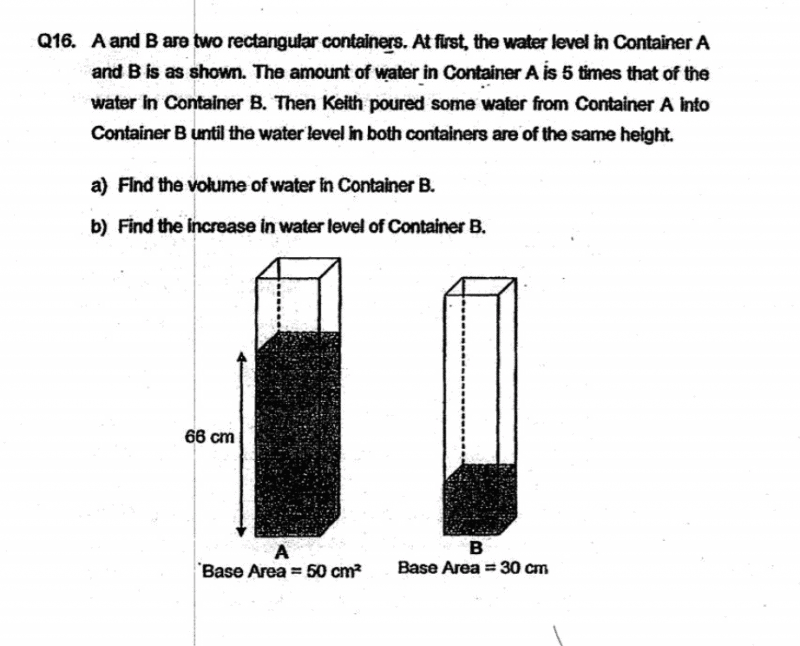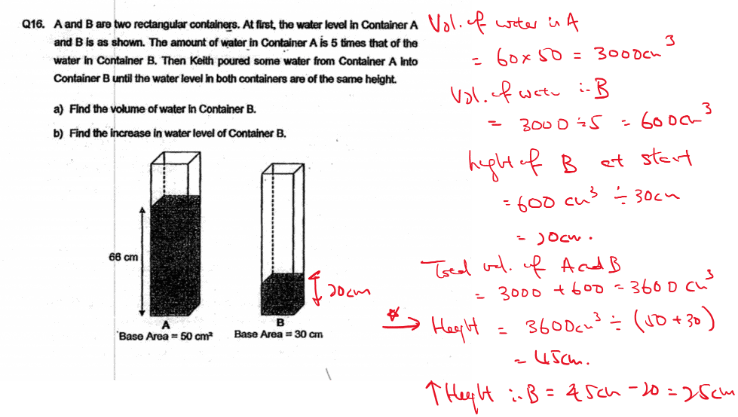# QuestionThanks in advance…

1 Answer

# AnswerHello this is typical question on change of water level which has several questions in PSLE so do guide your kid along in the understanding part of it.

The key in solving this question is where the height of both containers the same. Hence we can calculate the volume of both containers if they are only 1 cm in height. That will be 800 cm3. And we know the total volume in both A and B which is 3600 cm3. Hence I can find the height of both containers since they are the same.

This is an application of proportion method which Enso Education focuses on. Do check us up at here to know more about it!

0 Replies 1 Like ✔Accepted Answer